## Vector Exam1 and Problem Solutions

Vectors Exam1 and Problem Solutions

1. Find A+B+C.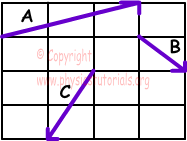First, we find A+B then add it to vector C.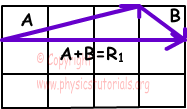We find R1, now we add C to R1 to find resultant vector.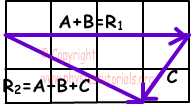R2=A+B+C

2.  Find resultant vector.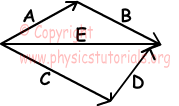Since; A+B=E and C+D=E

R=A+B+C+D+E

R=E+E+E=3E

3. A and A+2B vectors are given below. Find vector B.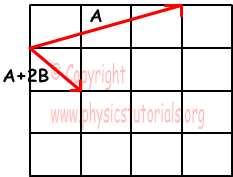A+2B-A=2B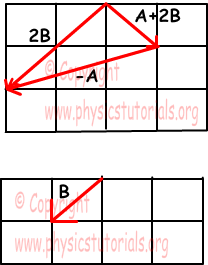To get vector B, we multiply 2B with 1/2.

4. Find resultant vector.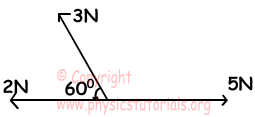F1+F2=5-2=3N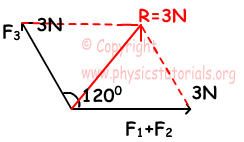F1+F2+F3=R=3N

5. Which one of the following statements is true?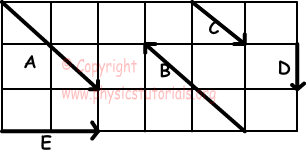I. A=B in magnitude

II. A=2C

III. E=2D

IV. A=B

As you can see in the figure given above, A and B are equal in magnitude, so I. is true. If you multiply C with 2, you get A, this means that II. is also true E =2D in magnitude but not in direction. Thus; III. is false.

6. If α3<α2<α1 and R1=R2=R3, find the relation between F1, F2 and F3.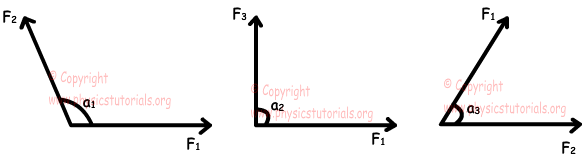Decreasing in the angle between forces increases the resultant force.

If α1=α2, then R1>R2 and F2>F3

If α2=α3, then R2>R3 and F1>F2

F1>F2>F3

7. Resultant vector of K, L and M is zero.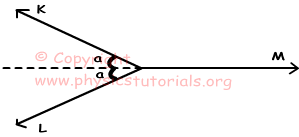Which one of the following statements given below is definitely false?

I. Ky and Ly components are equal vectors

II. K+L=M

III. α=600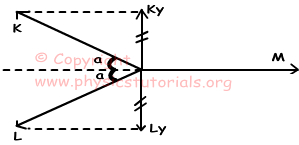I. Ky=-Ly, they are equal in magnitude but opposite in directions, thus they are not equal vectors.I. is false.

II. Magnitude of K+L=M, but directions are opposite, thus II is also wrong.

III: α=600 is possible.III is not exactly false.

Author:

Tags: Vectors |